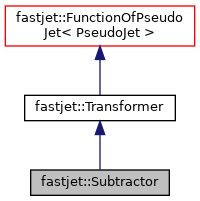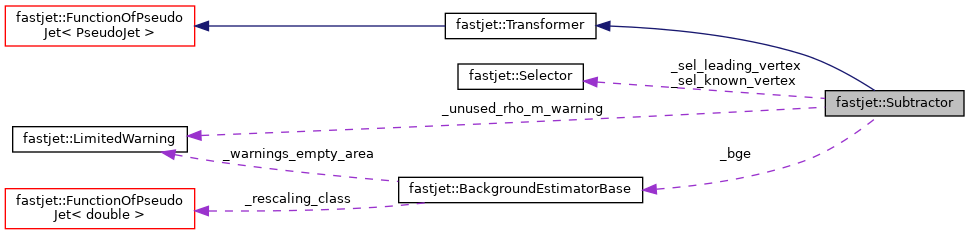FastJet  3.3.4
fastjet::Subtractor Class Reference

`#include <fastjet/tools/Subtractor.hh>`

Inheritance diagram for fastjet::Subtractor:[legend]
Collaboration diagram for fastjet::Subtractor:[legend]

## Public Member Functions

Subtractor (BackgroundEstimatorBase *bge)
define a subtractor based on a BackgroundEstimator

Subtractor (double rho)
define a subtractor that uses a fixed value of rho, the background pt density per unit area (which must be positive)

Subtractor (double rho, double rho_m)
define a subtractor that uses a fixed value of rho and rho_m; both must be >= 0;

Subtractor ()
default constructor

virtual ~Subtractor ()
default dtor

configuring the behaviour
void set_defaults ()
reset all parameters to default values More...

void set_use_rho_m (bool use_rho_m_in=true)
when 'use_rho_m' is true, include in the subtraction the correction from rho_m, the purely longitudinal, particle-mass-induced component of the background density per unit area More...

bool use_rho_m () const
returns whether or not the rho_m component is used

void set_safe_mass (bool safe_mass_in=true)
when 'safe_mass' is true, ensure that the mass of the subtracted 4-vector remain positive More...

bool safe_mass () const
returns whether or not safety tests on the mass are included

void set_known_selectors (const Selector &sel_known_vertex, const Selector &sel_leading_vertex)
This is mostly intended for cherge-hadron-subtracted type of events where we wich to use vertex information to improve the subtraction. More...Public Member Functions inherited from fastjet::Transformer
Transformer ()
default ctor

virtual ~Transformer ()
default dtorPublic Member Functions inherited from fastjet::FunctionOfPseudoJet< PseudoJet >
FunctionOfPseudoJet ()
default ctor

virtual ~FunctionOfPseudoJet ()
default dtor (virtual to allow safe polymorphism)

PseudoJet operator() (const PseudoJet &pj) const
apply the function using the "traditional" () operator. More...

std::vector< PseudoJetoperator() (const std::vector< PseudoJet > &pjs) const
apply the function on a vector of PseudoJet, returning a vector of the results. More...

## description and action

BackgroundEstimatorBase_bge
the tool used to estimate the background if has to be mutable in case its underlying selector takes a reference jet

double _rho
the fixed value of rho and/or rho_m to use if the user has selected that option

double _rho_m

bool _use_rho_m
include the rho_m correction

bool _safe_mass
ensures that the subtracted mass is +ve

Selector _sel_known_vertex
selects the particles with a known vertex origin

amongst the particles with a known vertex origin, select those coming from the leading vertex

static const double _invalid_rho = -numeric_limits<double>::infinity()
a value of rho that is used as a default to label that the stored rho is not valid for subtraction. More...

static LimitedWarning _unused_rho_m_warning

virtual PseudoJet result (const PseudoJet &jet) const
returns a jet that's subtracted More...

virtual std::string description () const
class description

PseudoJet _amount_to_subtract (const PseudoJet &jet) const
compute the 4-vector that should be subtracted from the given jetPublic Types inherited from fastjet::Transformer
typedef PseudoJetStructureBase StructureType
A typedef that is needed to ensure that the PseudoJet::structure_of() template function works.

## Detailed Description

Class that helps perform jet background subtraction.

This class derives from Transformer and makes use of a pointer to a BackgroundEstimatorBase object in order to determine the background in the vicinity of a given jet and then subtract area*background from the jet. It can also be initialised with a specific fixed value for the background pt density.

# Input conditions

The original jet must have area support (4-vector)

# Output/structure

The underlying structure of the returned, subtracted jet (i.e. constituents, pieces, etc.) is identical to that of the original jet.

Definition at line 62 of file Subtractor.hh.

## ◆ set_defaults()

 void fastjet::Subtractor::set_defaults ( )

reset all parameters to default values

Note: by default, the rho_m term is not included and the safety test for the mass is not done. This is mostly for backwards compatibility with FastJet 3.0 and is highly likely to change in a future release of FastJet

Definition at line 61 of file Subtractor.cc.

## ◆ set_use_rho_m()

 void fastjet::Subtractor::set_use_rho_m ( bool use_rho_m_in = `true` )
inline

when 'use_rho_m' is true, include in the subtraction the correction from rho_m, the purely longitudinal, particle-mass-induced component of the background density per unit area

Note: this will be switched off by default (for backwards compatibility with FastJet 3.0) but is highly likely to change in a future release of FastJet

Definition at line 102 of file Subtractor.hh.

## ◆ set_safe_mass()

 void fastjet::Subtractor::set_safe_mass ( bool safe_mass_in = `true` )
inline

when 'safe_mass' is true, ensure that the mass of the subtracted 4-vector remain positive

when true, if the subtracted mass is negative, we return a 4-vector with 0 mass, pt and phi from the subtracted 4-vector and the rapidity of the original, unsubtracted jet.

Note: this will be switched off by default (for backwards compatibility with FastJet 3.0) but is highly likely to change in a future release of FastJet

Definition at line 122 of file Subtractor.hh.

## ◆ set_known_selectors()

 void fastjet::Subtractor::set_known_selectors ( const Selector & sel_known_vertex, const Selector & sel_leading_vertex )
inline

This is mostly intended for cherge-hadron-subtracted type of events where we wich to use vertex information to improve the subtraction.

Given the following parameters:

Parameters
 sel_known_vertex selects the particles with a known vertex origin sel_leading_vertex amongst the particles with a known vertex origin, select those coming from the leading vertex Momentum identified as coming from the leading vertex will be kept, momentum identified as coming from a non-leading vertex will be eliminated and a regular area-median subtraction will be applied on the 4-vector sum of the particles with unknown vertex origin.

When this is set, we shall ensure that the pt of the subtracted 4-vector is at least the pt of the particles that are known to come from the leading vertex (if it fails, subtraction returns the component that is known to come from the leading vertex — or, the original unsubtracted jet if it contains no particles from the leading vertex). Furthermore, when safe_mass() is on, we also impose a similar constraint on the mass of the subtracted 4-vector (if the test fails, the longitudinal part of the subtracted 4-vector is taken from the component that is known to come from the leading vertex).

Definition at line 153 of file Subtractor.hh.

## ◆ result()

 PseudoJet fastjet::Subtractor::result ( const PseudoJet & jet ) const
virtual

returns a jet that's subtracted

Parameters
 jet the jet that is to be subtracted
Returns
the subtracted jet

Implements fastjet::Transformer.

Definition at line 72 of file Subtractor.cc.

## ◆ _invalid_rho

 const double fastjet::Subtractor::_invalid_rho = -numeric_limits::infinity()
staticprotected

a value of rho that is used as a default to label that the stored rho is not valid for subtraction.

Definition at line 203 of file Subtractor.hh.

The documentation for this class was generated from the following files: